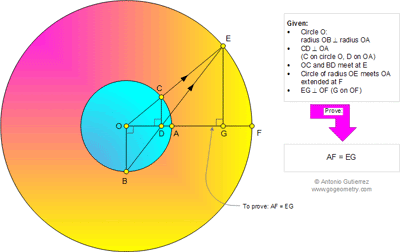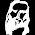## Monday, February 17, 2014

### Geometry Problem 985: Circle, Radius, Perpendicular, Concentric Circles, Congruence, Triangle

Geometry Problem. Post your solution in the comments box below.
Level: Mathematics Education, High School, Honors Geometry, College.

Click the figure below to see the complete problem 985.#### 2 comments:

1.1. CD/OA = EG/OF
2. CD/OA = CD/OB = CE/OE = AF/OF

Thus, AF=EG.

2.Let R, r denote the radii of the outer and inner concentric circles.
Now EG / r = EG / OB = DG / OD = EG / OB = CE / OC = AF / r
since AF = OF - OA = R - r = OE - OC = CE.
Hence EG = AF